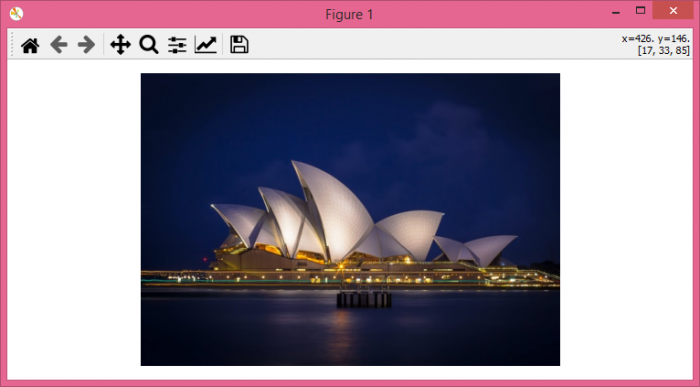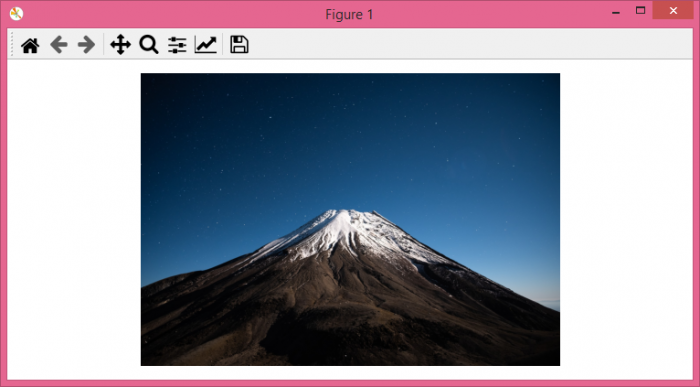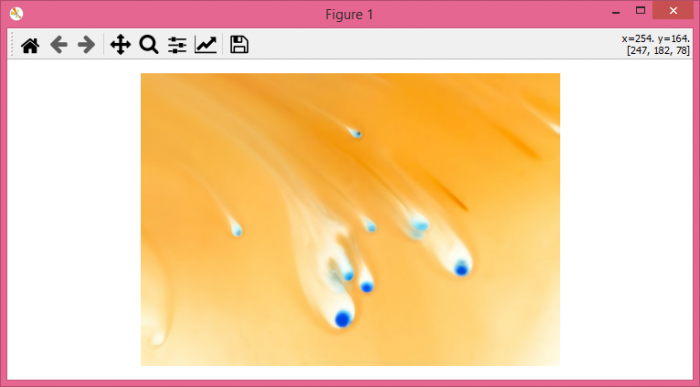# How to display a sequence of images using Matplotlib?

MatplotlibPythonData Visualization

To display a sequence of images using Matplotlib, we can take the following steps −

• Set the figure size and adjust the padding between and around the subplots.

• Make a list of images that have to be drawn.

• Turn off the axes.

• Iterate the images and redraw over the axes.

• Take a pause after each draw.

## Example

import matplotlib.pyplot as plt

plt.rcParams["figure.figsize"] = [7.50, 3.50]
plt.rcParams["figure.autolayout"] = True

images = ['opera.jpg', 'mountain.jpg', '9.jpg']
plt.axis('off')
img = None

for f in images:
if img is None:
img = plt.imshow(im)
plt.pause(0.5)
else:
img.set_data(im)
plt.pause(0.5)
plt.draw()

## Output

When we execute the code, it will show the three images that we supplied, one by one.